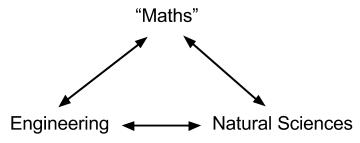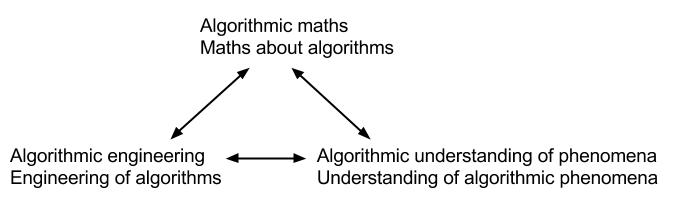What is Computer Science?
By Bo (back to homepage)

Computer Science is the study of algorithms: step-by-step procedures for solving problems or accomplishing tasks.

We can think of algorithms just as computer programs, but CS is far broader than writing programs! It encompasses the scientific study of algorithms: What are their fundamental capabilities and limitations? It also deals with the algorithmic study of science: What can we learn in, say, Biology, or Microeconomics, or Physics or Mathematics or more, by taking an algorithmic perspective?

Brief list of topics:
• Designing algorithms to solve problems (ex "Google maps": find the shortest route from A to B on a map).
• "Complexity" or difficulty of problems and relationships between them (ex "Fedex delivery": find the shortest route that visits all of the many delivery locations; and "Subset sum": given a list of numbers, pick out a bunch that sum up to 100; both problems seem hard and turn out to be "equivalent" in a cool way).
• Machine learning of facts or procedures from data (ex "OCR": train a comp to read handwritten zipcodes on letters).
• Building systems of software (or hardware) (examples all around us).
• What is intelligence and how to make algorithms that act "intelligently" in a complex situation (ex self-driving cars).
• ... and much more!

For fun and for the sake of argument, let's divide what we call science into three branches:By "Maths", I essentially mean deductive reasoning: formal definitions, theorems, and proofs. Engineering, of course, consists of building things to accomplish tasks; and the natural sciences seek to understand, explain, and predict phenomena in the real world. Of course, the branches all overlap and interact to different extents.

When we add the algorithmic perspective, we get computer science:Each aspect of science plays two roles in computer science: Looking in and looking out. Looking inwards at the nature of computation itself, we can: seek to prove things about algorithms; design or construct algorithms; and examine algorithmic phenomena in the real world. Looking outwards at other fields through the lens of computation, we can seek an algorithmic perspective on questions already studied in other fields.

Some examples:
• Maths, in: Theoretical CS: complexity, cryptography, algorithms, and so on. Much of CS not considered "theory" also fits here.
• Maths, out: Again much TCS: e.g. constructive/algorithmic (especially efficient) approaches to existential mathematics (examples: constructive Johnson-Lindenstrauss Lemma, efficiently computable codes, ....). Algorithmic Game Theory: computational approaches to problems and models in microeconomics.
• Engineering, in: Construction of algorithms; systems, programming languages, machine learning, software engineering.
• Engineering, out: Use of computer modeling, simulations, numerical analysis, etc. in building e.g. aeroplanes, cars, etc; making tools and devices "smarter" by giving them a brain (navigation, medical, etc).
• Natural sciences, in: Machine learning and systems: When do the algorithms we build perform well or poorly, and why? How do they perform on real data sets?
• Natural sciences, out: Modeling natural phenomena and algorithms for inference from collected data. e.g. wildly successful Markov-Chain Monte Carlo in biology, overlap of learning and statistics in e.g. econometrics.